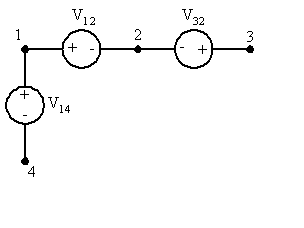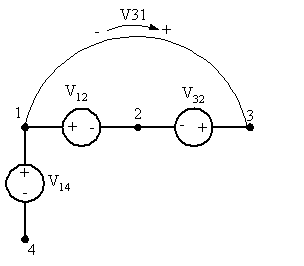# Phasor to complex units

• DODGEVIPER13

## Homework Statement

Several of the voltages associated with a certain circuit are given by V12=9∠30° V, V32=3∠132° V, V14=2∠10° V. Determine V21, V13, V34, and V24.

## The Attempt at a Solution

I am just using this problem as an example as I have no idea how to go from phasor to complex. So V13=V12+V32=9∠30°+3∠132°=? I don't really know here I can only imagine Magnitude*cos(θ)=Real and Magnitude*sin(θ)=imaginary I know this but how do I ultilize these beacuse everything I have done does not work?

Your first example is magnitude 9, phase angle 30 degrees. So the complex number is 9 x [cos(30) + j sin(30)]; this is what you have proposed. Make sure your calculator/computer is set to degrees, or else convert them to radians.

The alternative expression (in radians) may be more useful: use the Euler identity and write it as
9 exp(j*30/360*2pi).

Ok thanks man

hey for V13 I get 5.865+j6.798 V

back in phasor I get 8.978∠49.21°

V21=V32+V13=3.936+j9.096

V34=V13+V14=7.834+j7.145 and V24=V32+V34=5.906+j9.443

V21=V32+V13=3.936+j9.096

As a reality check, note that you are given a value for V12. Shouldn't V21 = -V12?

oh heh so it should be V21=-9∠30° V

Also are the rest of my answers correct?

oh heh so it should be V21=-9∠30° V

Right. So if the method you employed previously doesn't result in that value, something may be amiss with your method. Better check into that.

Note that it's common practice to roll the "negative" into the angle in order to leave the magnitude positive. Add + or - 180° to the angle.

To keep things straight I'd suggest creating a little circuit fragment containing the "known" points and their given potential differences. Something like:The voltage supply polarities reflect the subscript convention, namely Vab would be the potential at a with respect to b, so that by convention a is where your meter's "+" lead would go and b would be where the "-" lead would go, if you were to make the measurement Vab. The actual values assigned to the supplies may be positive or negative depending upon what you're given.

Then, to find any potential difference between points, perform the "KVL walk" between them and sum the potential changes.

#### Attachments

so for V13=V12+V32

so for V13=V12+V32

By convention V13 would be the potential at 1 with respect to 3. So start at 3 and "walk" to 1. Pay attention to the polarities of the sources along the way.

Ahhh woops V13=V12-V32

V34=sqrt((V14)^2+(V32)^2+(V12)^2) right?

or should it be V34=sqrt(-(V14)^2+(V32)^2+(V12)^2)

hmmmmmmm V31=V32-V12 so that V34=V31-V41

V34=sqrt((V14)^2+(V32)^2+(V12)^2) right?

What are you trying to accomplish? The individual voltages are in complex form and you can sum them by summing their real and imaginary components separately.

If you want the magnitude of the result, then do the square root of the sum of the squares of its components.

so I solved V13=9.801+j2.271

I was trying to use Pythagorean Theorem which I know is incorrect sorry

did you read my new post after that is that close to how to solve for V34

more specifically post #18

did you read my new post after that is that close to how to solve for V34

more specifically post #18

I think you're still tripping over the directions of the potential changes. If you calculate a new potential difference, like V31, add a new branch to the diagram with the appropriate label and source direction. You can just use arrows to indicate polarity if the diagram starts getting too cluttered.So for V34 you would start at V4 and walk through +V14 and +V31, right?

#### Attachments

ok well V31=V32-V12=-9.801-j2.271

then V34=V14+V31

-7.831-j1.923=V34 hopefully?

-7.831-j1.923=V34 hopefully?

That looks okay.

V24=-V12+v14 = -5.824-j4.152